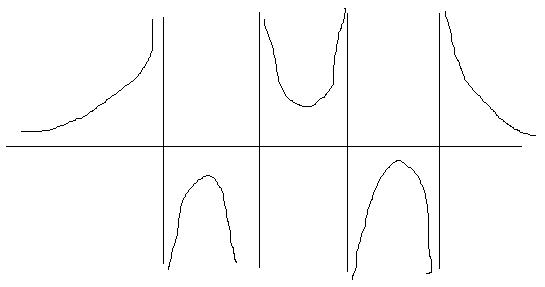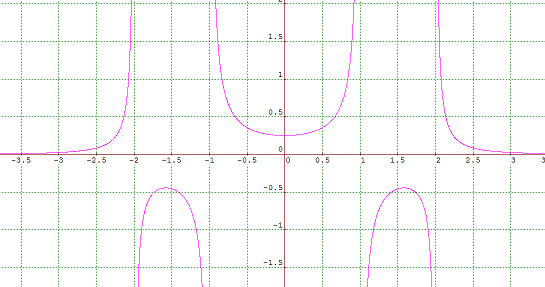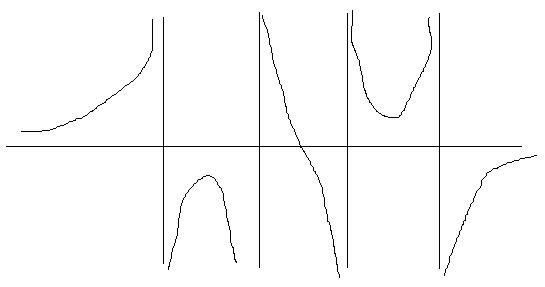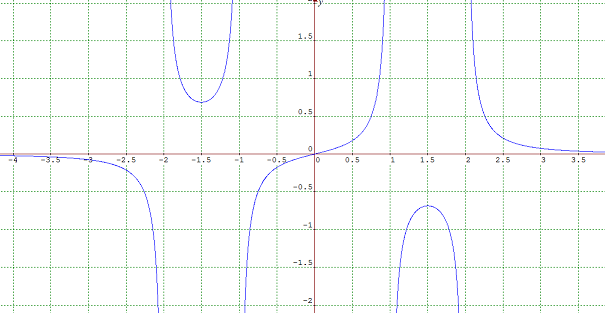#### You may also like### Quartics

Investigate the graphs of y = [1 + (x - t)^2][1 + (x + t^)2] as the parameter t varies.### Sine Problem

In this 'mesh' of sine graphs, one of the graphs is the graph of the sine function. Find the equations of the other graphs to reproduce the pattern.### Cocked Hat

Sketch the graphs for this implicitly defined family of functions.

# Rational Request

##### Age 16 to 18 Challenge Level:

Steve said the following

I sketched four vertical asymptotes and a sketch showed that a function which decayed to zero from above at $x \rightarrow \pm \infty$ could have the right sorts of properties.To get the right asymptotes and behaviour at $\pm \infty$ I guessed the following curve, choosing to make it symmetric about the origin for simplicity
$$y = \frac{1}{(x-2)(x-1)(x+1)(x+2)}$$
This worked: it has a turning point at $x$ between $-2$ and $-1$ another turning point at $x$ between $1$ and $2$ and a turning point at $x=0$.

The plot of this from graphmatica is as followsIt seems likely that many such curves, with differing constants, would also give the correct behaviour. To see why, upon differentiation, I get a cubic polynomial divided by another polynomial. For zeros the numerator would need to be zero and a cubic can have three real roots. I could choose the constants to have the correct number of real roots.

I then considered the second request. Initially, I thought that this seemed impossible, but then started to work through the possibilities for asymptotes. By turning the middle turning point into a point of inflection I would have a graph with the correct behaviour.I wondered how to convert the behaviour of the central turning point and decided that the curve needed to be forced to pass through the origin and also to be antisymmetric. I therefore multiplied the expression by $x$, realising that this wouldn't affect the 'topological' behaviour at the other turning points. A plot of the curve
$$y = \frac{x}{(x-2)(x-1)(x+1)(x+2)}$$
gave graphWhich has the correct behaviour.